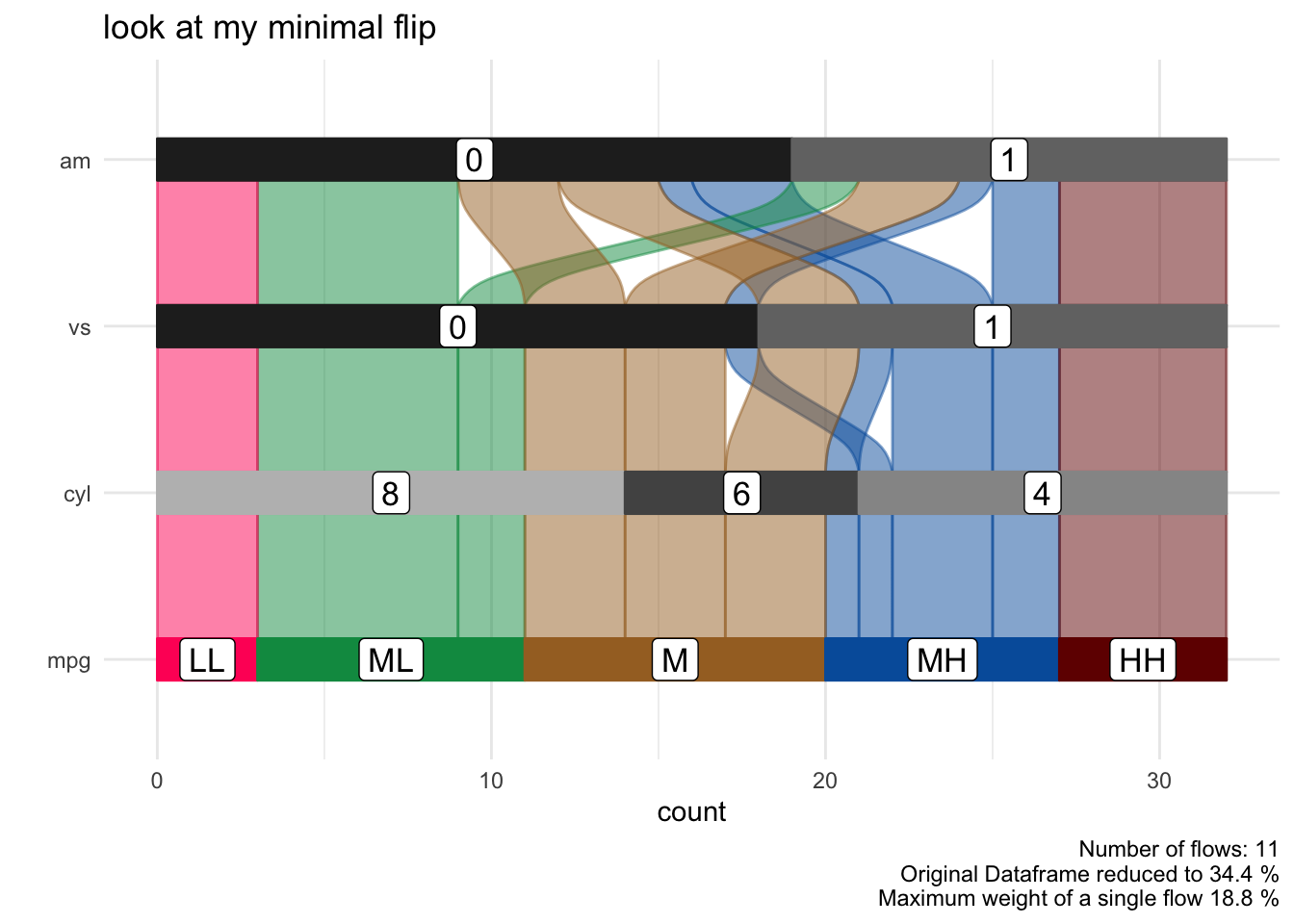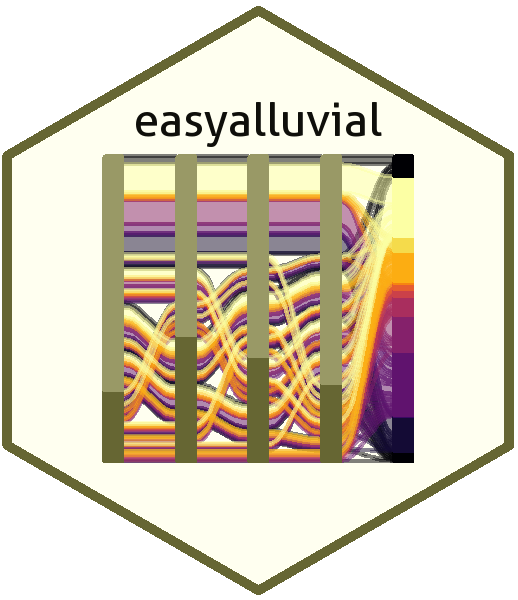# Introduction

Alluvial plots are a form of sankey diagrams that are a great tool for exploring categorical data. They group categorical data into flows that can easily be traced in the diagram. Other than sankey diagrams they are constrained to x-y dimensions, however their graphical grammar is a bit mor complex then that of a regular x-y plot. The `ggalluvial` package made a great job of translating that grammar into ggplot2 synthax and gives you many option to tweak the appearance of a plot, nevertheless there still remains a multilayered complexity that makes it difficult to use `ggalluvial` for explorative data analysis. `easyalluvial` provides a simple interface to this package that allows you to put out a decent alluvial from any dataframe where data is stored in either long or wide format while automatically binning continuous data. It is meant to allow a quick visualisation of entire dataframes similar to the visualisations created by the `tabplot` package providing different colouring options which give it the flexibility needed for data exploration.

# easyalluvial

github repository

## Features

• plot alluvial graph with a single line of code of a given datafram
• support for wide and long data format (wiki, wide vs. long/narrow data)
• automatically transforms numerical to categorical data
• helper functions for variable selection
• convenient parameters for coloring and ordering

## Install

``````# install.packages("devtools")
devtools::install_github("erblast/easyalluvial")``````

## Wide Format

### Sample data

``````suppressPackageStartupMessages( require(easyalluvial) )
suppressPackageStartupMessages( require(tidyverse) )``````
``````data_wide = as_tibble(mtcars)
categoricals = c('cyl', 'vs', 'am', 'gear', 'carb')
numericals = c('mpg', 'cyl', 'disp', 'hp', 'drat', 'wt', 'qsec')

data_wide = data_wide %>%
mutate_at( vars(categoricals), as.factor ) %>%
mutate( car_id = row_number() )``````
``````## Note: Using an external vector in selections is ambiguous.
## ℹ Use `all_of(categoricals)` instead of `categoricals` to silence this message.
## ℹ See <https://tidyselect.r-lib.org/reference/faq-external-vector.html>.
## This message is displayed once per session.``````
``knitr::kable( head(data_wide) )``
mpg cyl disp hp drat wt qsec vs am gear carb car_id
21.0 6 160 110 3.90 2.620 16.46 0 1 4 4 1
21.0 6 160 110 3.90 2.875 17.02 0 1 4 4 2
22.8 4 108 93 3.85 2.320 18.61 1 1 4 1 3
21.4 6 258 110 3.08 3.215 19.44 1 0 3 1 4
18.7 8 360 175 3.15 3.440 17.02 0 0 3 2 5
18.1 6 225 105 2.76 3.460 20.22 1 0 3 1 6

### alluvial_wide()

#### Binning of Numerical Variables

This function produces a simple alluvial plot of the given dataframe. Numerical variables are centered, scaled and yeo johnson transformed (transformed to resemble more of a normal distribution) by `easyalluvial::manip_bin_numerics()`. Outliers as defined by the boxplot criteria ( see documentation for `grDevices::boxplot.stats()`) are stunted to to the min and max values that are defined by the whiskers of the box plot. The so transformed numeric variables are then cut into 5 (default) equally ranged bins which are lebeled ‘LL’ (low-low), ‘ML’ (medium-low), ‘M’ (medium), ‘MH’ (medium-high), HH (high-high) by default.

``````alluvial_wide(data_wide
, bins = 5 # Default
, bin_labels = c('LL','ML','M','MH','HH') # Default
, fill_by = 'all_flows'
)``````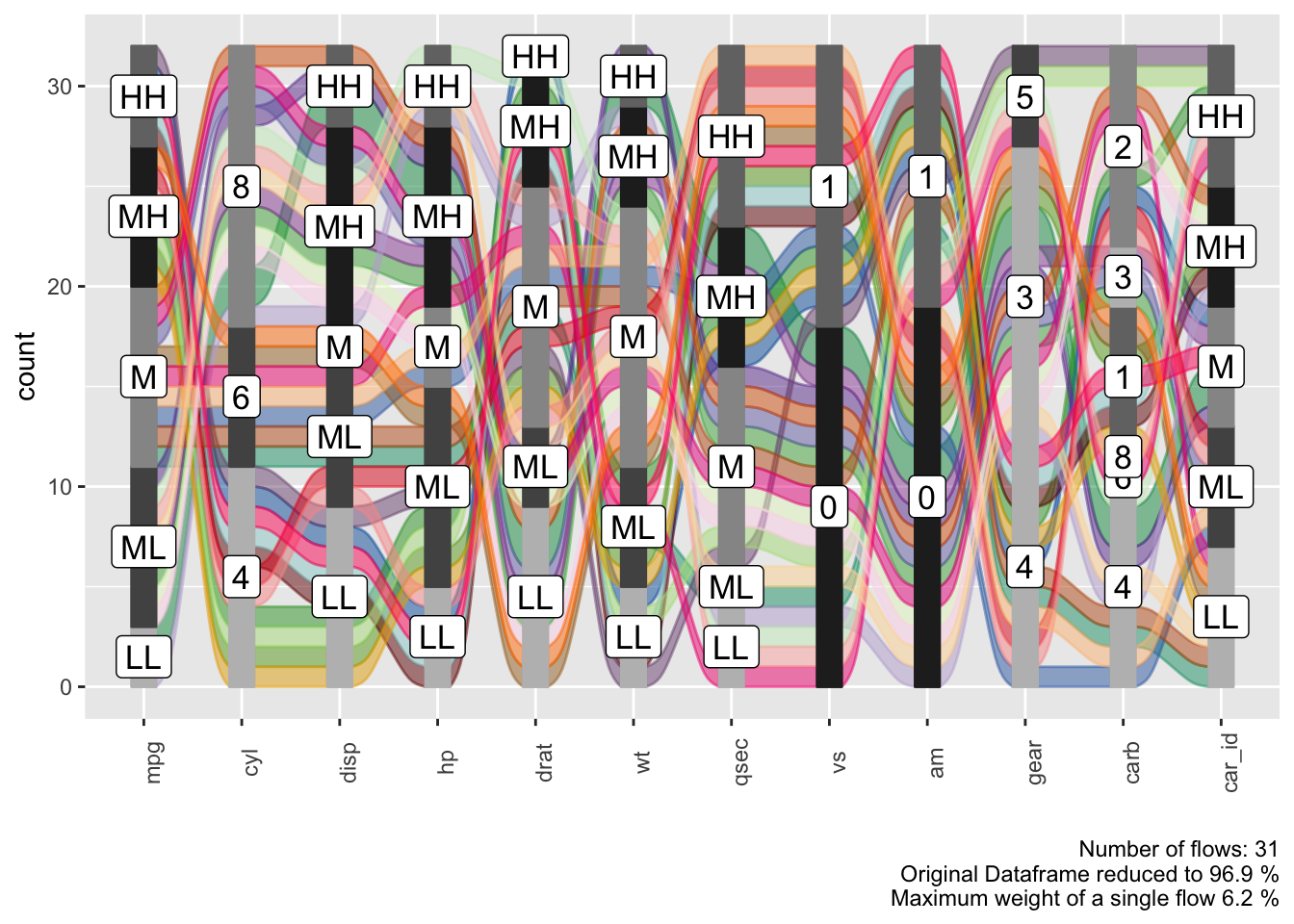#### Alluvial Plot Organisation

Each group of stacked bars represents a variable while the size of each segment represents how many observations in the dataframe belong to that level machting the segment label. The colored flows between the bar stack represent a group of observations that match the value for each variable indicated by the flow. The thickness of the flow indicates how many observations belong to that group.

We see that each flow has more or less the same thickness and the statistical information in the plot caption tells us that we have 30 flows in total for 32 observations in the dataframe. Which means that almost each observation is unique in its combination of variable values.

#### Reduce the Number of Variables

In order to reduce the compexity we can use a helper function `plot_condensation()` to get an idea which variables we want to include in our alluvial plot. Lets say we are especially interested in the `mpg` (miles per gallon) variable and how it relates to the other variables. `plot_condensation` will look for other variables it can combine `mpg` with while trying to condense the data frame to a minimum.

``plot_condensation(data_wide, first = mpg)``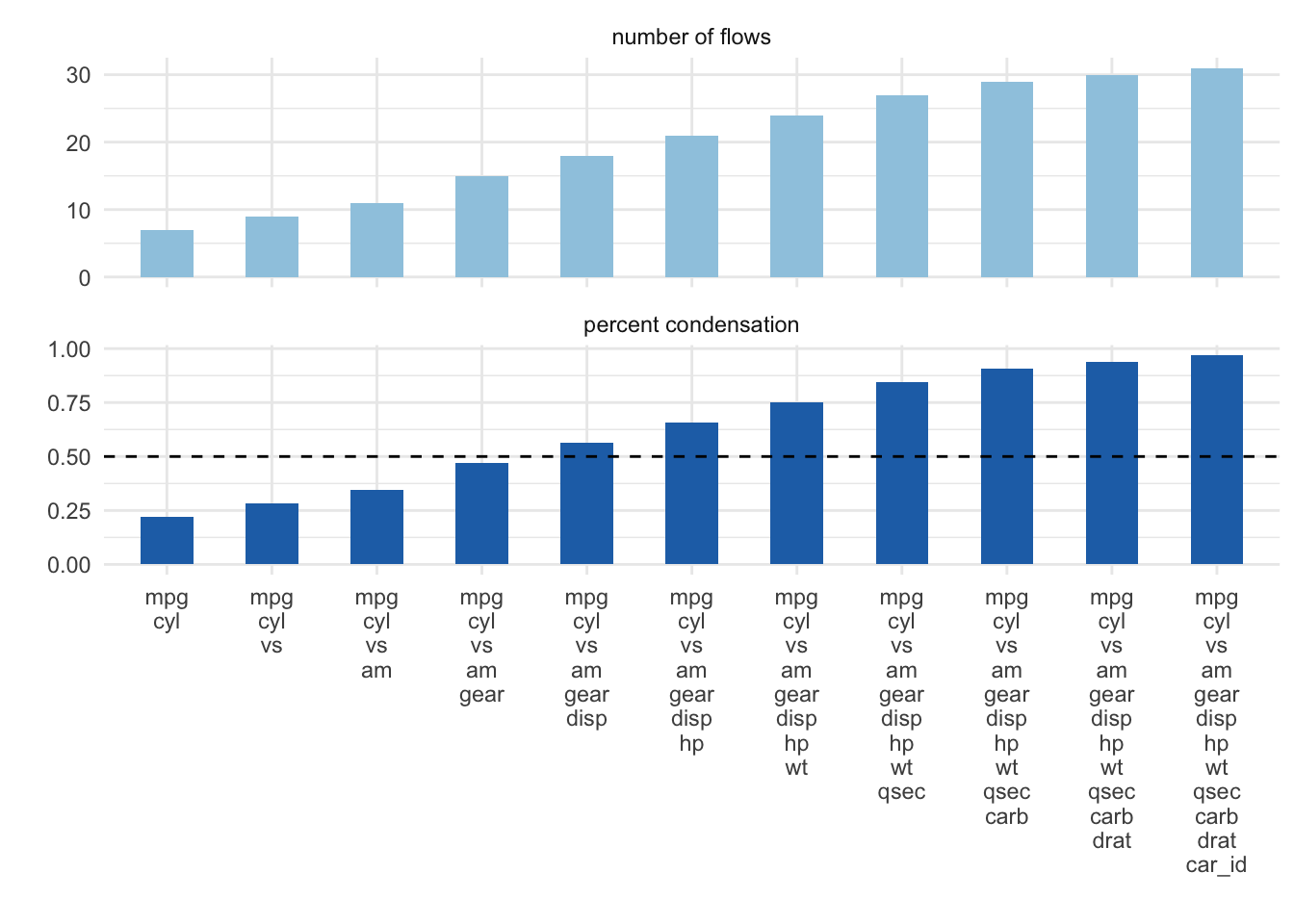In general we want to condense the dataframe to 50% or less we might get a meaningfull alluvial when looking at `mpg, cyl, vs, am` in that order.

``alluvial_wide( select(data_wide, mpg, cyl, vs, am), fill_by = 'first_variable' )``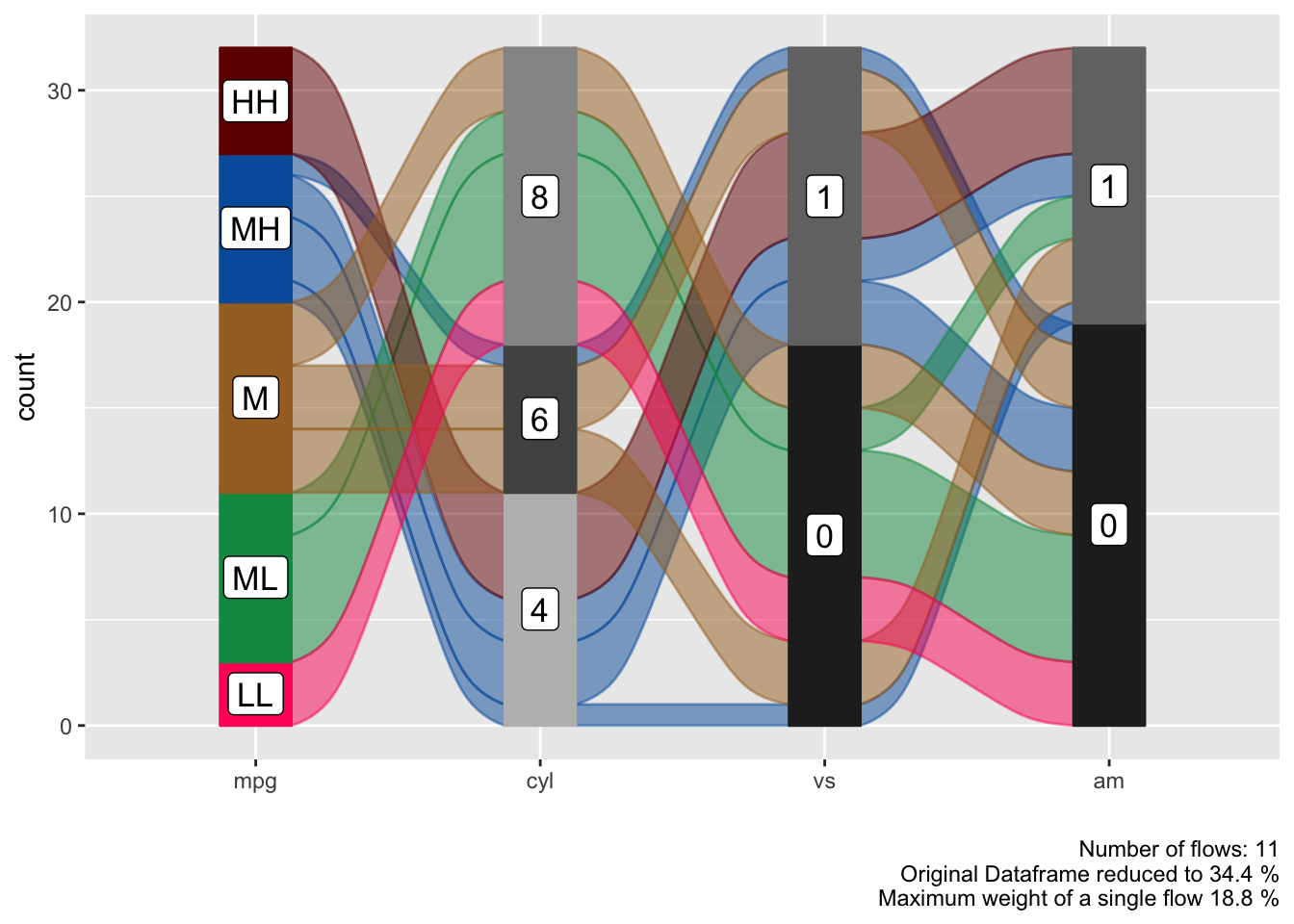#### Reorder Levels

We can see a clear pattern in the flows now, especially now that we have colored the flows by the `mpg` variable. However some of the flows are unnecessarily criss-crossing. We can improve this by changing the order of the levels of the `cyl` variable.

``````alluvial_wide( select(data_wide, mpg, cyl, vs, am)
, fill_by = 'first_variable'
, order_levels = c('8','6','4') )``````If levels of several variables have levels of the same name we cannot order them individually per variable, this is a design choice (see documentation of `alluvial_wide()` ). If we want to reorder them we need to assign individual level names first.

``````p_unordered = alluvial_wide( select(data_wide, mpg, disp, hp)
, fill_by = 'first_variable' ) +
labs( title = 'Unordered', caption = '\n\n' )

bin_labels = c('mpg_LL','mpg_LM','mpg_M','mpg_MH','mpg_HH')

p_ordered = data_wide %>%
mutate( mpg = manip_bin_numerics(mpg, bin_labels = bin_labels)
, mpg = fct_rev(mpg) ) %>%
select( mpg, disp, hp) %>%
alluvial_wide() +
labs( title = 'Ordered')

gridExtra::grid.arrange( p_unordered, p_ordered, nrow = 1 )``````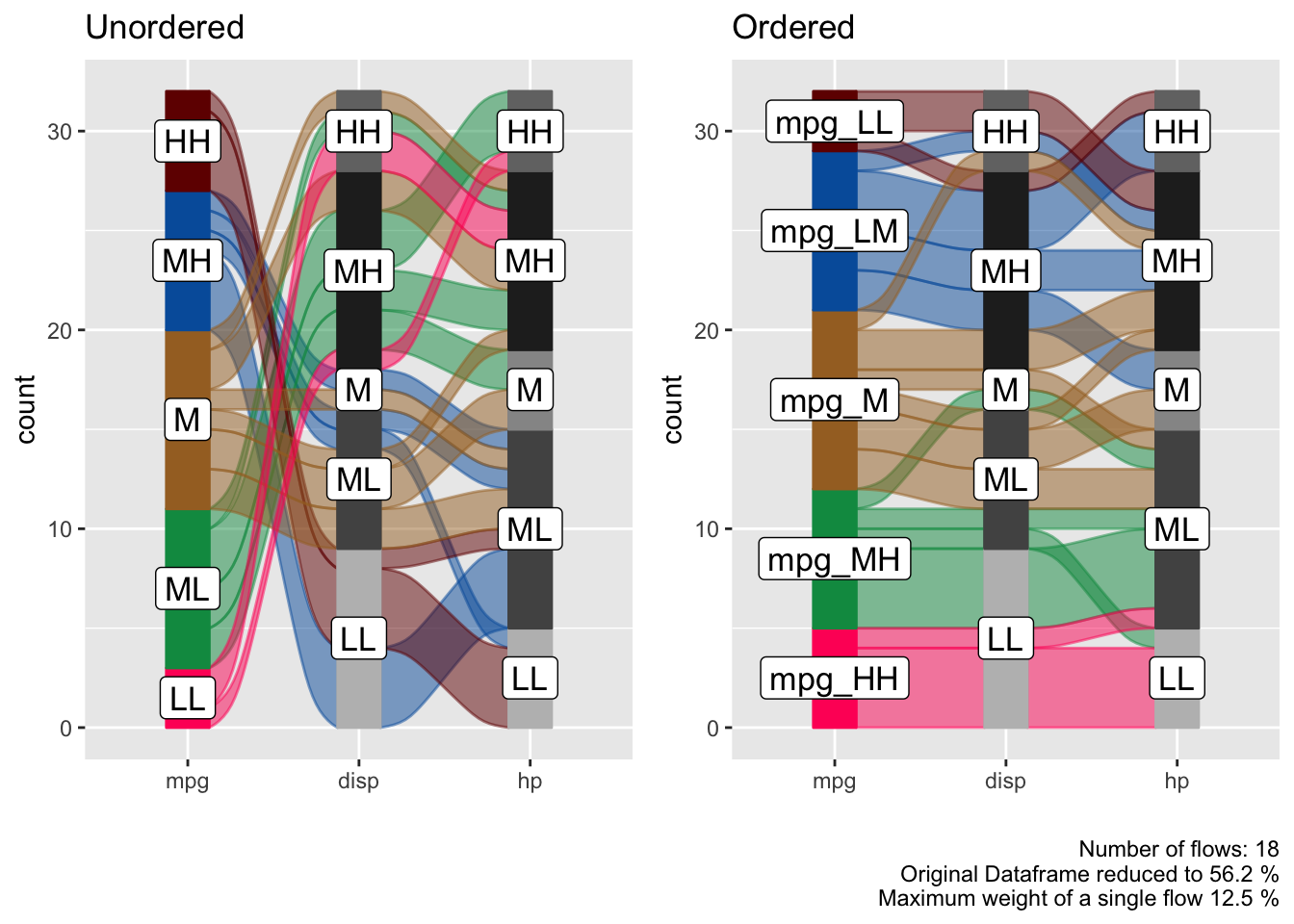## Long Format

In certain cases we might want to start with a dataframe that is already in long format, this is mostly the case for time-series data where we want to track a categorical value over different time periods.

### Sample Data

``````monthly_flights = nycflights13::flights %>%
group_by(month, tailnum, origin, dest, carrier) %>%
summarise() %>%
group_by( tailnum, origin, dest, carrier) %>%
count() %>%
filter( n == 12 ) %>%
select( - n ) %>%
left_join( nycflights13::flights ) %>%
.[complete.cases(.), ] %>%
ungroup() %>%
mutate( flight_id = pmap_chr(list(tailnum, origin, dest, carrier), paste )
, qu = cut(month, 4)) %>%
group_by(flight_id, carrier, origin, dest, qu ) %>%
summarise( mean_arr_delay = mean(arr_delay) ) %>%
ungroup() %>%
mutate( mean_arr_delay = ifelse( mean_arr_delay < 10, 'on_time', 'late' ) )``````
``## Joining, by = c("tailnum", "origin", "dest", "carrier")``
``````  levels(monthly_flights\$qu) = c('Q1', 'Q2', 'Q3', 'Q4')

data_long = monthly_flights

flight_id carrier origin dest qu mean_arr_delay
N0EGMQ LGA BNA MQ MQ LGA BNA Q1 on_time
N0EGMQ LGA BNA MQ MQ LGA BNA Q2 on_time
N0EGMQ LGA BNA MQ MQ LGA BNA Q3 on_time
N0EGMQ LGA BNA MQ MQ LGA BNA Q4 on_time
N11150 EWR MCI EV EV EWR MCI Q1 late
N11150 EWR MCI EV EV EWR MCI Q2 late

### alluvial_long()

In long format we only need the column that contains the keys (timepoints, Q1, Q2, Q3, Q4) and the values (late, ontime), but we also need a column for the ID (flight_id) because in long format data for one flight is spread over 4 rows and the function needs to know which IDs to group to put into a flow. If ther is implicitly missing data so one flight_id has less than in this case four rows of data (one for each timepoint) it will be made explicit and labelled `'NA'`.

``````col_vector = c('tomato', 'green3')

alluvial_long(data_long
, key = qu
, value = mean_arr_delay
, id = flight_id
, fill_by = 'value'
, col_vector_flow = col_vector
, col_vector_value = col_vector
)``````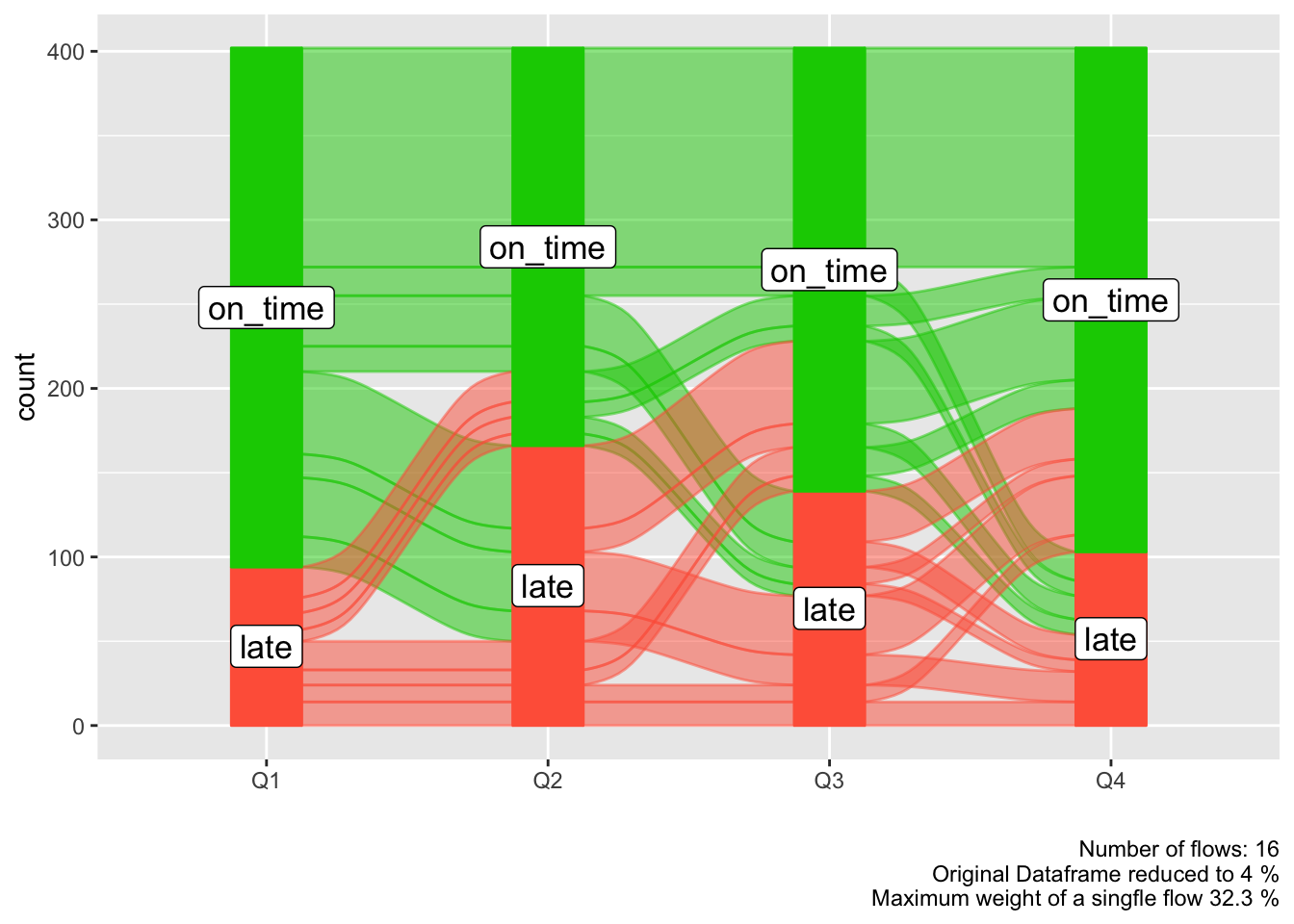We might be more interested which airline carrier had the most late flights, we can add it as an additional variable to the plot and use it for coloring. We can place this variable either on the left or on the right.

``````p_right = alluvial_long(data_long
, key = qu
, value = mean_arr_delay
, id = flight_id
, fill = carrier
, fill_by = 'last_variable'
, fill_right = T # Default
) +
labs(title = 'Color fill variable placed to the right')

p_left = alluvial_long(data_long
, key = qu
, value = mean_arr_delay
, id = flight_id
, fill = carrier
, fill_by = 'last_variable'
, fill_right = F
) +
labs(title = 'Color fill variable placed to the left'
, caption = '\n\n')

gridExtra::grid.arrange( p_left, p_right, nrow = 1)``````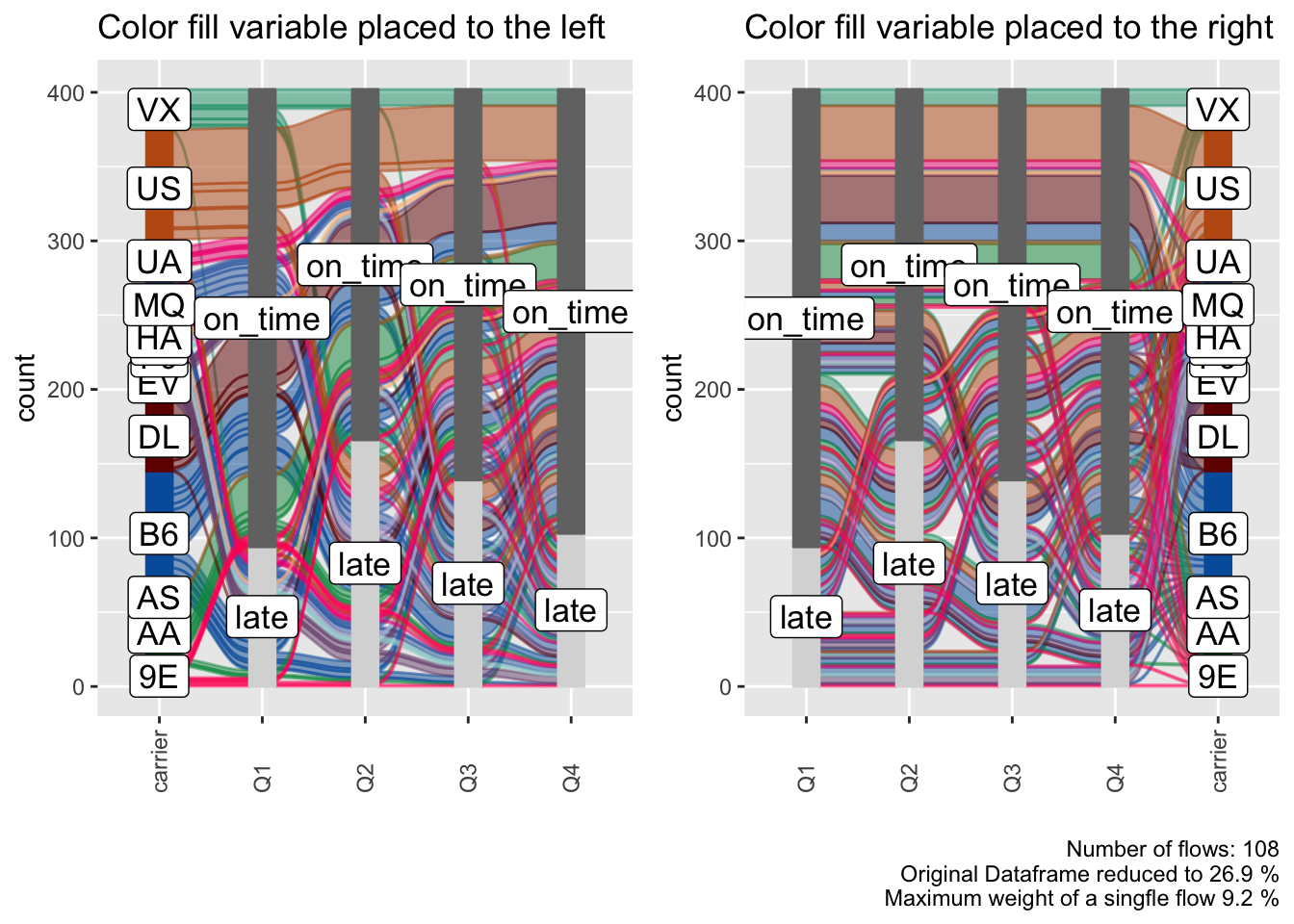#### Order Levels

`alluvial_long()` takes three different `order_levels_*` arguments, one for the keys (x-axis) one for the values (y-axis) and one for the fill variable. Here we want to demonstrate how to order the `carrier` variable by number of flights.

``````carriers_ordered_by_count = data_long %>%
group_by(carrier) %>%
count() %>%
arrange( n ) %>%
.[['carrier']]

alluvial_long(data_long
, key = qu
, value = mean_arr_delay
, id = flight_id
, fill = carrier
, fill_by = 'last_variable'
, order_levels_fill = carriers_ordered_by_count
, fill_right = F
) +
labs(title = 'Carriers ordered by number of flights')``````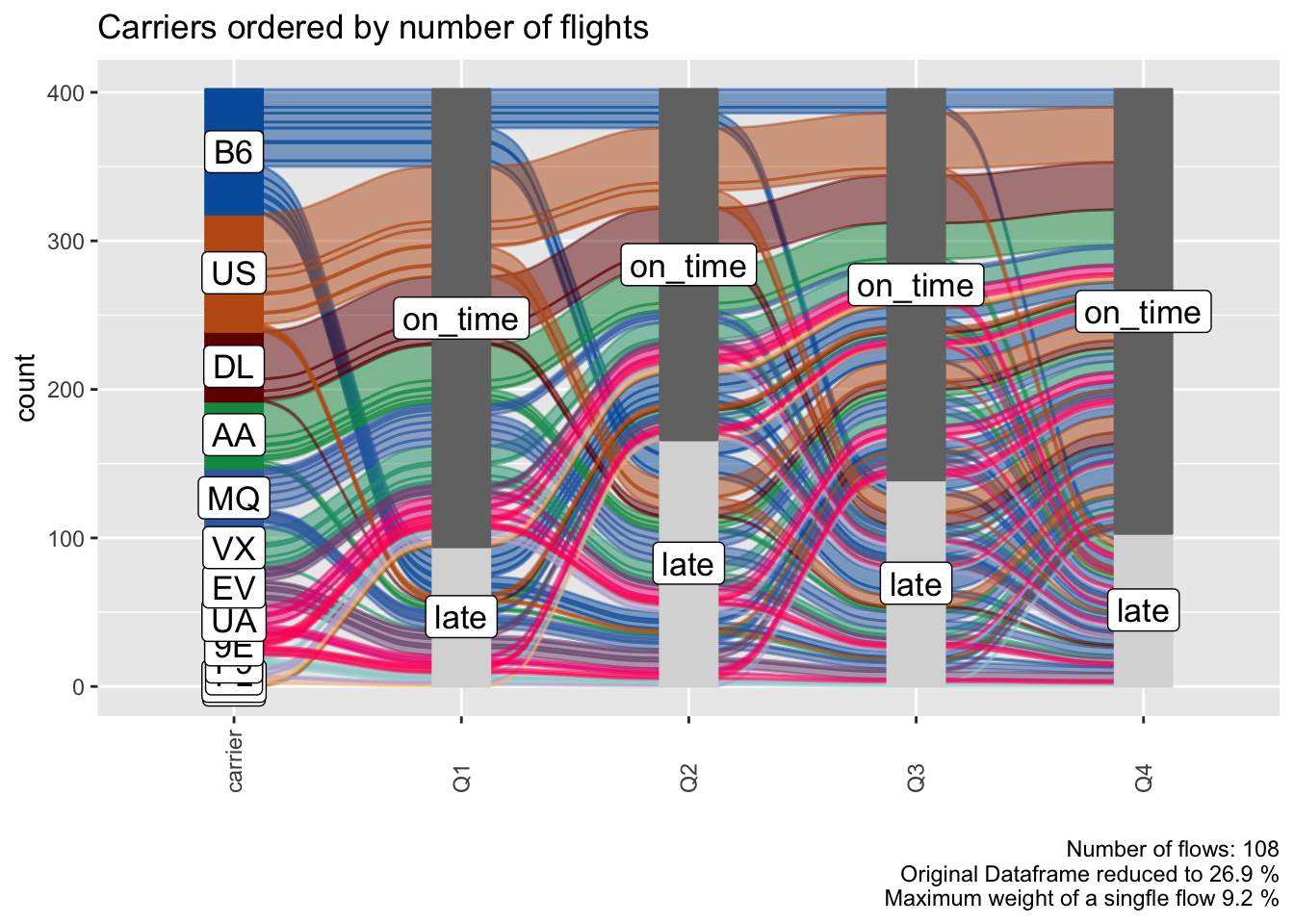## General

### Missing Data

Explicitly and implicitly missing data will automaticall be labeled as `'NA'` and added as a level. The order of that level can be changed like any other. We will automatically generate implicitly missing data if we sample only a fraction of our long format dataframe, because then not every flight_id will have a value (late, on_time) assigned for each time point (Q1, Q2, Q3, Q4). We can replace `'NA'` with any other string.

``````col_vector = c( 'tomato', 'grey', 'green3')

data_na = data_long %>%
select(flight_id, qu, mean_arr_delay) %>%
sample_frac(0.9)

alluvial_long(data_na
, key = qu
, value = mean_arr_delay
, id = flight_id
, fill_by = 'value'
, NA_label = 'None'
, col_vector_value = col_vector
, col_vector_flow = col_vector
) +
labs(title = 'Implicit NA values')``````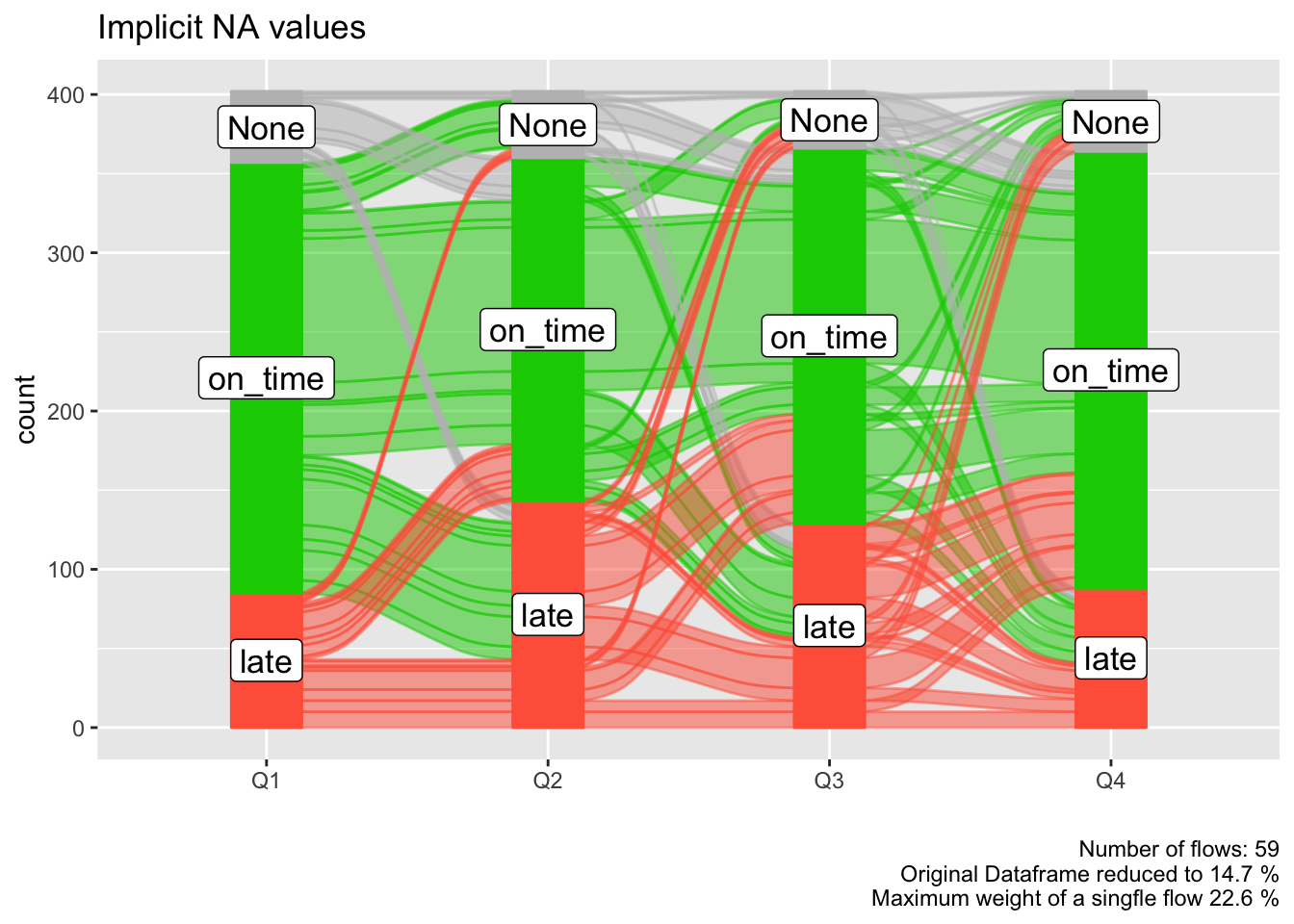### Colors

`alluvial_wide()` and `alluvial_long` take any sequence of either HEX code or string color values. `easyalluvial` offers some convenience functions around constructing qualitative color pallettes for distinct values.

``````palette_qualitative() %>%
palette_filter(greys = F) %>%
palette_plot_rgp()``````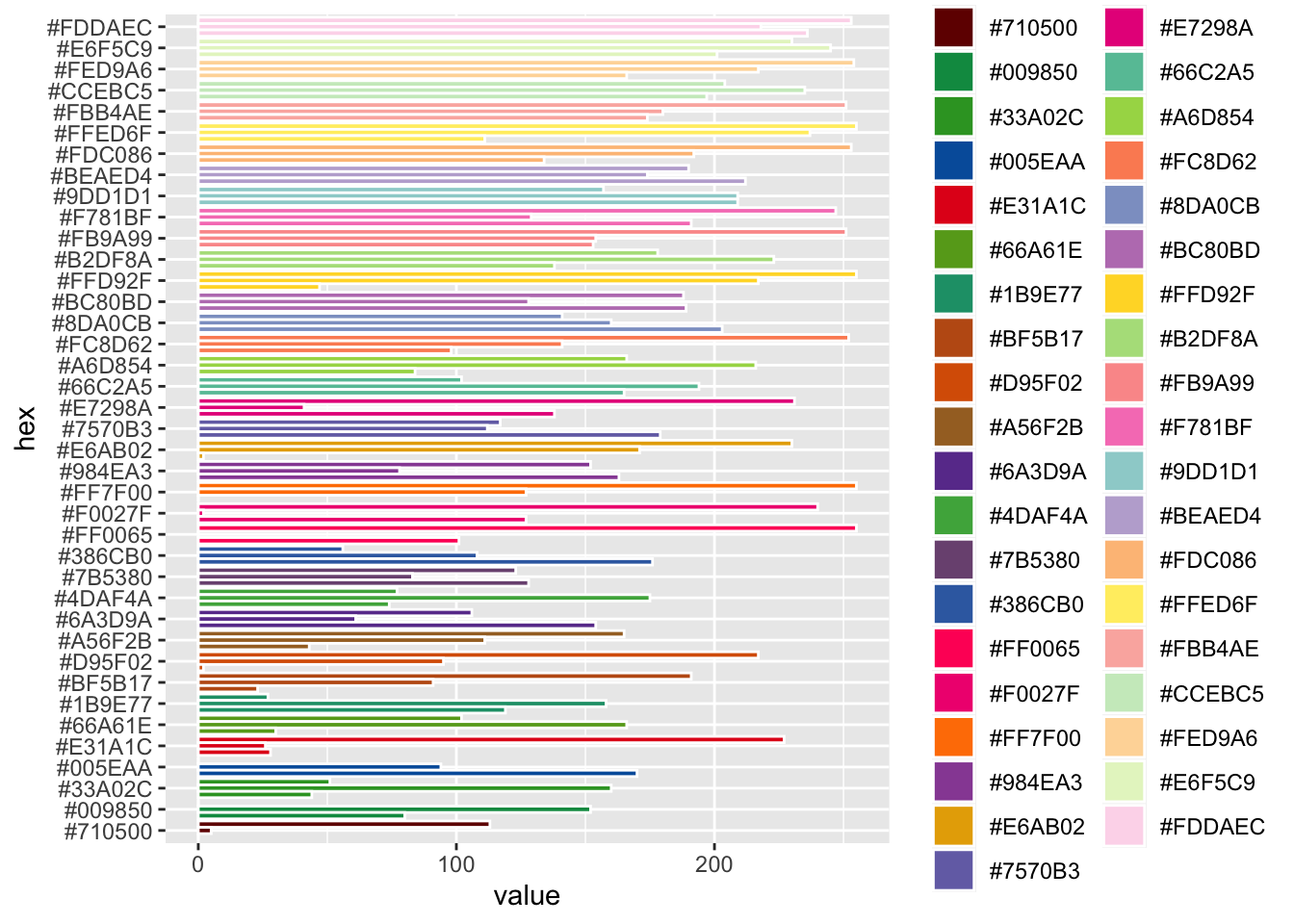### Connect Flows to observations in original data

We might recognise interesting patterns in the alluvial plot that we want to follow up upon. For example which cars With medium-low `mpg` and 8 `cyl` and 0 `vs` has an `am` value of 1. Note that we are passing the `car_id` variable.

``````p = alluvial_wide( select(data_wide, mpg, cyl, vs, am, car_id)
, id = car_id
, fill_by = 'first_variable'
, order_levels = c('8','6','4') )

p``````The plot objects returned by both functions have an attribute called `data_key` which is an x-y table arranged like the alluvial plot one column containing the original ID. We can use the `car_id` variable to rejoin the original dataframe.

``````p\$data_key %>%
filter( mpg == 'ML'
, cyl == 8
, vs == 0
, am == 1 ) %>%
# in order to convert factors to integers we have to convert them
# to character first. Converting from factor returns the order of
mutate( car_id = as.character(car_id)
, car_id = as.integer(car_id) ) %>%
left_join(data_wide, by = 'car_id') %>%
knitr::kable()``````
car_id mpg.x cyl.x vs.x am.x alluvial_id n mpg.y cyl.y disp hp drat wt qsec vs.y am.y gear carb
29 ML 8 0 1 11 2 15.8 8 351 264 4.22 3.17 14.5 0 1 5 4
31 ML 8 0 1 11 2 15.0 8 301 335 3.54 3.57 14.6 0 1 5 8

### ggplot2 manipulations

thanks to `ggalluvial` the alluvial plots that `easyalluvial` returns can be manipulated using `ggplot2` synthax

``````p +
coord_flip() +
theme_minimal() +
ggtitle('look at my minimal flip')``````